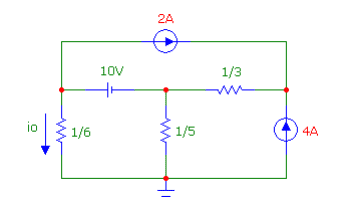# Thevenin Equivalent Circuit

• Engineering
• nobodyuknow

## Homework StatementAbove given is the circuit I am supposed to simplify where the 1/5Ω resistor is the load. I'm unsure how to convert this circuit into an equivalent Thevenin Circuit.

## Homework Equations

V = I*R (Ohm's Law)

## The Attempt at a Solution

Using Microcap and it's Dynamic DC Analysis Tool, I measured the current flow at the load. I managed to break it down to this:

http://img844.imageshack.us/img844/282/5x8t.png [Broken]

Where V5 was a 2/3V voltage source. (2A current source in series with the 1/3Ω resistor).

If this is correct, I would then say that the 2/3V voltage source and the 4A current source were in series and that the V4 voltage source and the 1/6Ω resistor were in series. Is this the correct approach?

Last edited by a moderator:
Remember that a Thevenin equivalent is a single voltage source with a single series resistance (for DC circuits).

What's the usual algorithm for finding the Thevenin equivalent analytically?

Would the first step be setting all those current sources to 0? Thus left with the remaining voltage source and the two resistors?

http://img820.imageshack.us/img820/2318/q2z4.png [Broken]

Last edited by a moderator:
No. You must suppress ALL sources to find the Thevenin resistance: Remove all current sources and short all voltage sources.

To find the Thevenin voltage you must find the open-circuit potential across the load (remove the load and find the potential across the open terminals).

Your course notes or text should cover this!